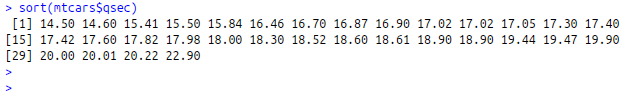• #5, First Floor, 4th Street Dr. Subbarayan Nagar Kodambakkam, Chennai-600 024 Landmark : Samiyar Madam
• pro@slogix.in
• +91- 81240 01111

### How to implement rank, order and sort function in R?

###### Description

To implement the rank, order and sort function using R programming.

#### Rank :

Returns a vector with the "rank" of each value

R Function :rank()

#### Order :

Returns the indices that would put the initial vector xin order.

Using order function to sort a data frame

R Function :order()

#### Sort :

To sort a vector into acsending or descending order

R Function :sort()

###### Sapmle Code

#Input Vector
vect<-c(92,22,42,34,66,85,46,36,84)
#Rank Function
rank(vect)

#Order Function
order(vect)
vect[order(vect)]

#Order Using mtcars dataset
attach(mtcars)
mtcars[order(mpg),]

#Sort Function
sort(mtcars\$qsec)

###### Screenshots